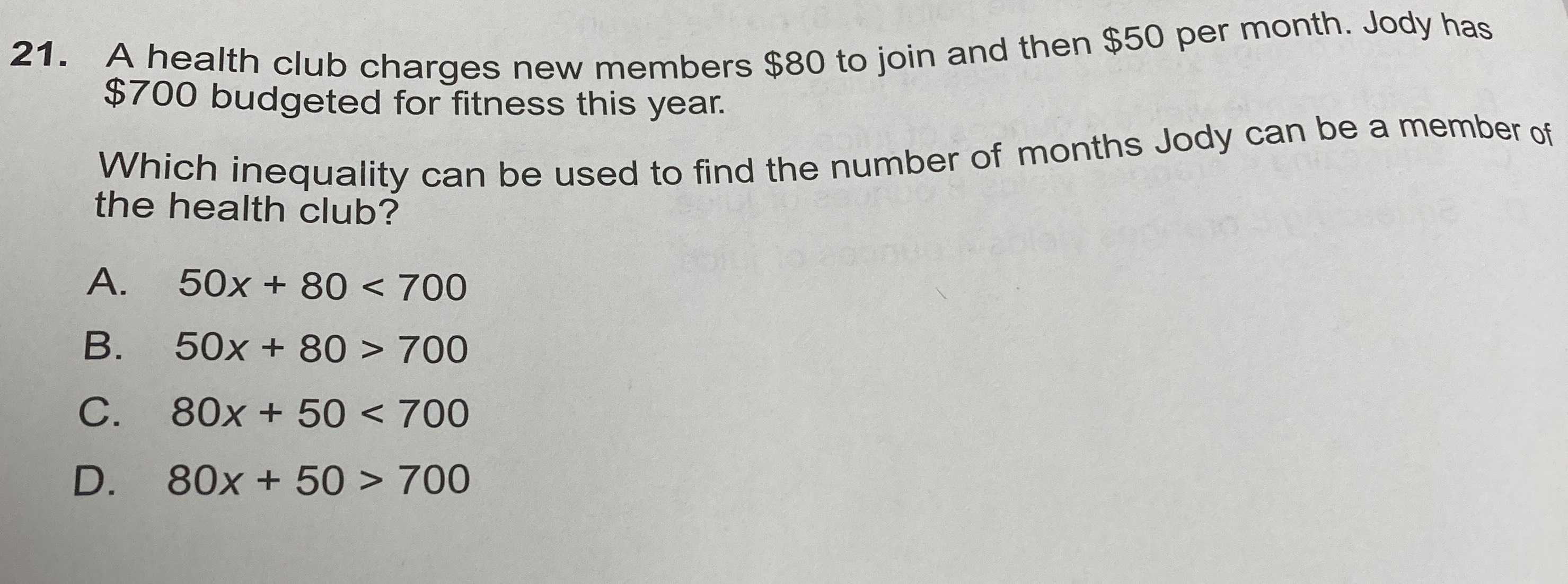### ¿Todavía tienes preguntas de matemáticas?

Pregunte a nuestros tutores expertos
Algebra
Pregunta21. A health club charges new members $$\ 80$$ to join and then $$\ 50$$ per month. Jody has

$$\ 700$$ budgeted for fitness this year. Which inequality can be used to find the number of months Jody can be a member of the health club? A. $$50 x + 80 < 700$$

B. $$50 x + 80 > 700$$

C. $$80 x + 50 < 700$$

D. $$80 x + 50 > 700$$